## RS Aggarwal Class 8 Solutions Chapter 18 Area of a Trapezium and a Polygon Ex 18A

These Solutions are part of RS Aggarwal Solutions Class 8. Here we have given RS Aggarwal Solutions Class 8 Chapter 18 Area of a Trapezium and a Polygon Ex 18A.

Other Exercises

Question 1.
Solution:
In trapezium ABCD,
Length of parallel sides
AB = 24 cm, DC = 20 cm
and distance between them = 15 cm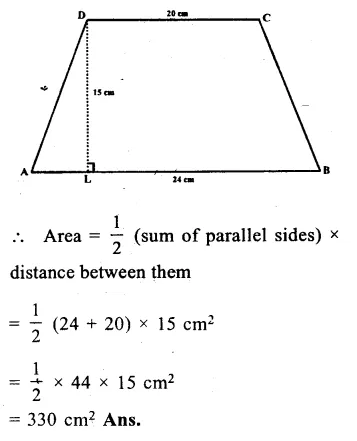Question 2.
Solution:
Parallel sides of a trapezium ABCD are
l1 = 38.7 cm. and l2 = 22.3 cmQuestion 3.
Solution:
Parallel sides of the trapezium = 1 m, 1.4 m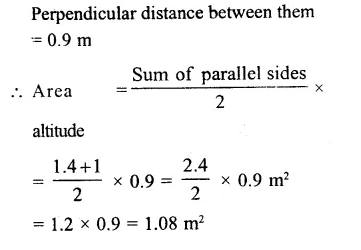Question 4.
Solution:
Area of trapezium = 1080 cm²
Lengths of parallel sides are
l1 = 55 cm and l2 = 35 cm
Let h be the distance between them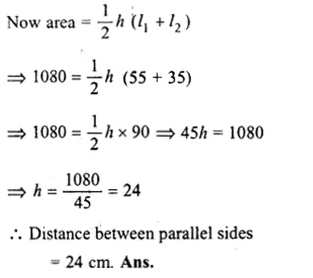Question 5.
Solution:
Area of trapezium shaped field = 1586m²
Distance between parallel sides = 26 m
Sum of the parallel sides = $$\frac { Area\times 2 }{ Altitude }$$
= $$\frac { 1586\times 2 }{ 26 }$$ = 122 m
One side = 84 m
Second side = 122 – 84
= 38 m

Question 6.
Solution:
Area of trapezium = 405 cm²
Ratio in parallel sides = 4:5
and distance between them = 18 cm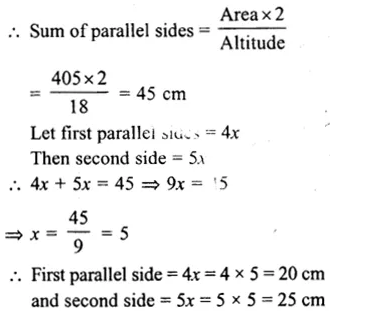Question 7.
Solution:
Area of trapezium = 180 cm²
Height (h) = 9 cm.
Let l1 and l2 be the parallel sides,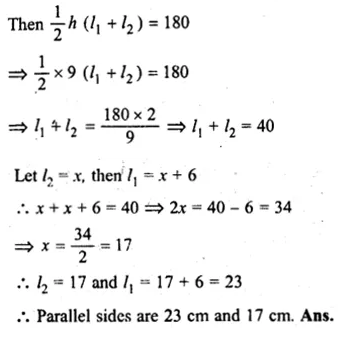Question 8.
Solution:
Let one of parallel sides = x
Then second sides = 2x
Area = 9450 m²
Distance between them = 84 m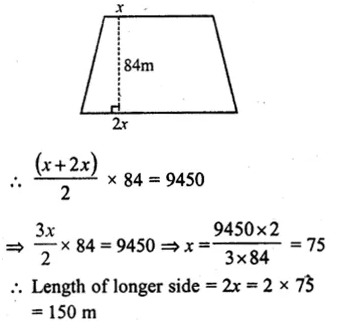Question 9.
Solution:
Perimeter of trapezium ABCD = 130 m
BC = 54 m, CD = 19 m, AD = 42 mQuestion 10.
Solution:
In the given trapezium ABCD, AC isQuestion 11.
Solution:
In trapezium ABCD,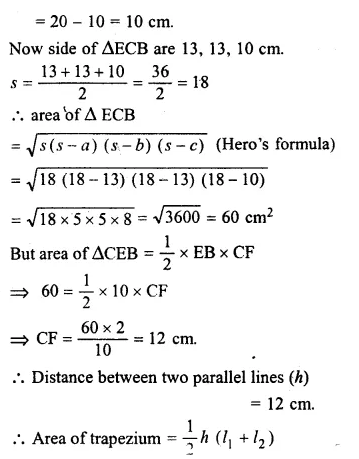Question 12.
Solution:
In trapezium ABCD,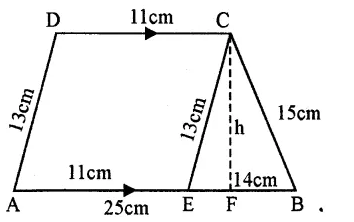AB || DC
AB = 25 cm DC = 1 cm
AD = 13 cm and BC = 15 cm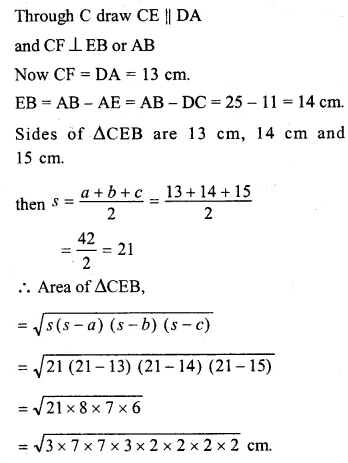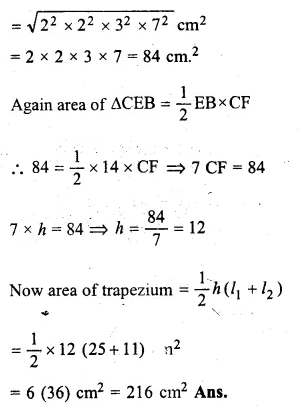Hope given RS Aggarwal Solutions Class 8 Chapter 18 Area of a Trapezium and a Polygon Ex 18A are helpful to complete your math homework.

If you have any doubts, please comment below. Learn Insta try to provide online math tutoring for you.